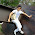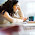## Binary Tree Preorder Traversal (iteration)

Given a binary tree, return the preorder traversal of its nodes' values.
For example:
Given binary tree {1,#,2,3},
   1
\
2
/
3

return [1,2,3].
Note: Recursive solution is trivial, could you do it iteratively?

### Analysis:

Classical problem,  the recursion version of the solution can be found here (you need do slight modification, can you do that?) . In this post, I solved it iteratively.  Which data structure do remind us ?  Yes! Stack!

The algorithm has following steps:
(1) Push the root node into the stack.
(2) while the stack is not empty, do:
a. pop the top node and print it.
b. push the right child of the top node (if exists)
c. push the left child of the top node (if exists)

### Code(C++):

/**
/**
* Definition for binary tree
* struct TreeNode {
*     int val;
*     TreeNode *left;
*     TreeNode *right;
*     TreeNode(int x) : val(x), left(NULL), right(NULL) {}
* };
*/
class Solution {
public:
vector<int> preorderTraversal(TreeNode *root) {
stack<TreeNode*> st;
vector<int> res;
if (!root){return res;}
st.push(root);
while (!st.empty()){
TreeNode* top = st.top();
res.push_back(top->val);
st.pop();
if (top->right!=NULL){st.push(top->right);}
if (top->left!=NULL){st.push(top->left);}
}
return res;
}
};


### Code(Python):

# Definition for a  binary tree node
# class TreeNode:
#     def __init__(self, x):
#         self.val = x
#         self.left = None
#         self.right = None

class Solution:
# @param root, a tree node
# @return a list of integers
def preorderTraversal(self, root):
res = []
st = []
if root != None:
st.append(root)
while len(st) != 0:
tmp = st.pop()
res.append(tmp.val)
if (tmp.right != None):
st.append(tmp.right)
if (tmp.left != None):
st.append(tmp.left)
return res



1.2.